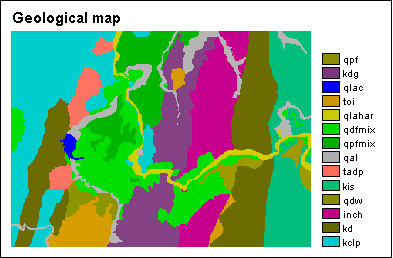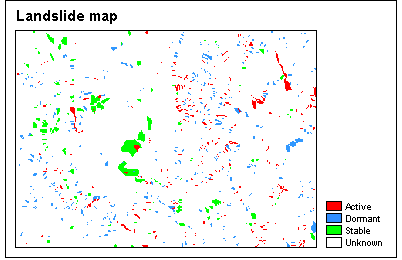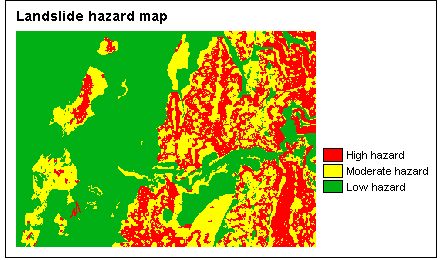# Summary

5. Statistical landslide hazard analysis

This exercise will show a method to make a hazard map based on quantitatively defined weight-values. Many different methods exist for the calculation of weight-values. The method used here is called the landslide index method.

The method is based on crossing a landslide map with some parameter maps. The cross operations return a number of cross tables; these are used to calculate the density of landslides per parameter class. For each parameter class, such as a lithological unit or a slope class, a weight-value is calculated as the natural logarithm of the landslide density in the class divided by the landslide density in the entire map. This ensures that weight values will be negative when the landslide density is lower than normal, and positive when it is higher than normal. Finally, you will create a hazard map.

Input data

In this exercise the landslide hazard map is made using only two parameter maps: a geological map and a slope map.

Furthermore, information on landslides is stored in a table; this table is linked to a map which depicts individual landslides. The table contains detailed information for each landslide (type, activity, depth, etc.).

The maps are from the Chinchiná area, department of Caldas, central Colombia.Calculating landslide density for each parameter classFirst, you will make an attribute map of the landslides: by using column Activity from the landslide table, you will obtain a map which depicts Active, Dormant or Stable landslides.

Subsequently, you will use the Cross operation twice:

• to cross the landslide map with the existing slope map
• to cross the landslide map with the existing geology map

The Cross operation allows you to know all occurring combinations of the input maps; the results are stored in a cross table. You will thus obtain two cross tables.

Next, you will open these tables, and use a number of TabCalc statements in order to calculate density values for active landslides:

• landslide density for the entire map,
• landslide densities per slope class, and
• landslide densities per geological unit.

From the cross table with the slope map, you will see that most active landslides occur on moderate slopes (20-30°), the highest density of active landslides is found on steep slopes (50-60°), there are no active landslides on very steep slopes (>70°).

Creating the weight maps

After some exercise in joining the calculated columns into a new table (to reduce redundant information), TabCalc is used to calculate weight factors for each slope class:

LN(density of active landslides per class / density of active landslides in entire map)

where LN is the natural logarithm.

As mentioned above:

• weight values will be negative when the landslide density is lower than normal, and
• weight values will be positive when the landslide density is higher than normal.Similarly you will calculate weight factors for the densities of active landslide per geological class.

Then, you will create two attribute maps, using the two calculated Weight columns. The first map thus contains weight factors regarding slide densities for slope classes; the second map contains weight factors regarding slide densities for geological units.

Creating the hazard maps

Finally, the landslide hazard map can be calculated using MapCalc: you will simply sum both weight maps.

With Slicing, you can then classify the resulting hazard map into three classes of low, moderate and high hazard. You will have to set the classify boundaries yourself. Depending on these boundaries, output results may be different.